BigPipe 是 Facebook 开发的优化网页加载速度的技术。网上几乎没有用 node.js 实现的文章，实际上，不止于 node.js，BigPipe 用其他语言的实现在网上都很少见。以至于这技术出现很久以后，我还以为就是整个网页的框架先发送完毕后，用另一个或几个 ajax 请求再请求页面内的模块。直到不久前，我才了解到原来 BigPipe 的核心概念就是只用一个 HTTP 请求，只是页面元素不按顺序发送而已。

``````{
"name": "bigpipe-experiment"
, "version": "0.1.0"
, "private": true
, "dependencies": {
"express": "3.x.x"
, "consolidate": "latest"
}
}
``````

app.js:

``````var express = require('express')
, cons = require('consolidate')
, path = require('path')

var app = express()

app.set('views', path.join(__dirname, 'views'))

app.use(function (req, res) {
res.render('layout', {
s1: "Hello, I'm the first section."
, s2: "Hello, I'm the second section."
})
})

app.listen(3000)
``````

``````doctype html

title Hello, World!
style
section {
margin: 20px auto;
border: 1px dotted gray;
width: 80%;
height: 150px;
}

section#s1!=s1
section#s2!=s2
``````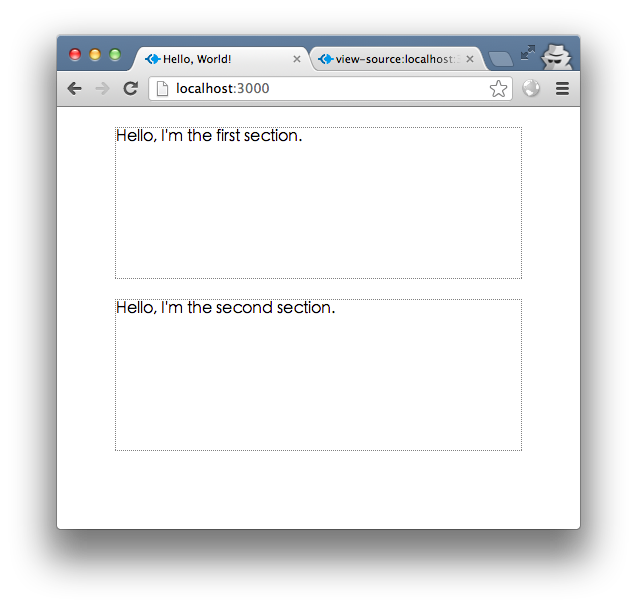``````h1 Partial 1
.content!=content
``````

``````h1 Partial 2
.content!=content
``````

``````section h1 {
font-size: 1.5;
margin: 0;
border-bottom: 1px dotted gray;
}
section div {
margin: 10px;
}
``````

``````var temp = {
}
app.use(function (req, res) {
res.render('layout', {
s1: temp.s1({ content: "Hello, I'm the first section." })
, s2: temp.s2({ content: "Hello, I'm the second section." })
})
})
``````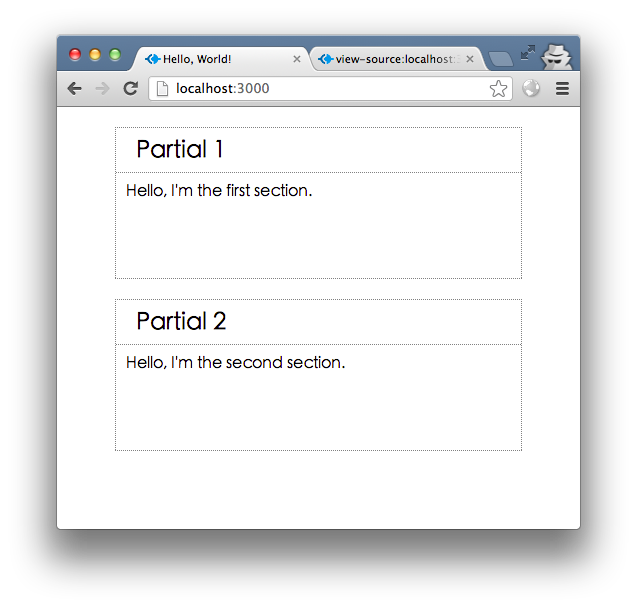``````var getData = {
d1: function (fn) {
setTimeout(fn, 3000, null, { content: "Hello, I'm the first section." })
}
, d2: function (fn) {
setTimeout(fn, 5000, null, { content: "Hello, I'm the second section." })
}
}
``````

``````app.use(function (req, res) {
getData.d1(function (err, s1data) {
getData.d2(function (err, s2data) {
res.render('layout', {
s1: temp.s1(s1data)
, s2: temp.s2(s2data)
})
})
})
})
``````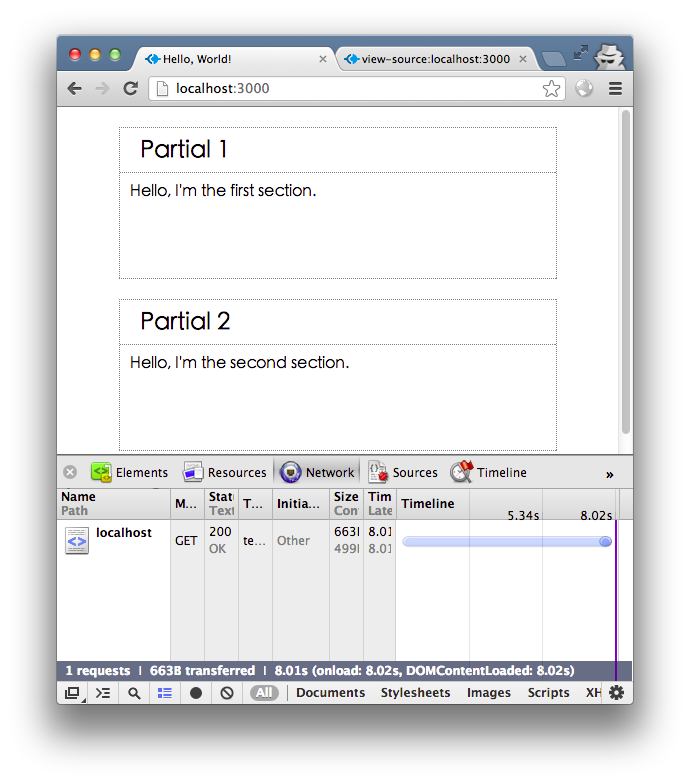``````app.use(function (req, res) {
var n = 2
, result = {}
getData.d1(function (err, s1data) {
result.s1data = s1data
--n || writeResult()
})
getData.d2(function (err, s2data) {
result.s2data = s2data
--n || writeResult()
})
function writeResult() {
res.render('layout', {
s1: temp.s1(result.s1data)
, s2: temp.s2(result.s2data)
})
}
})
``````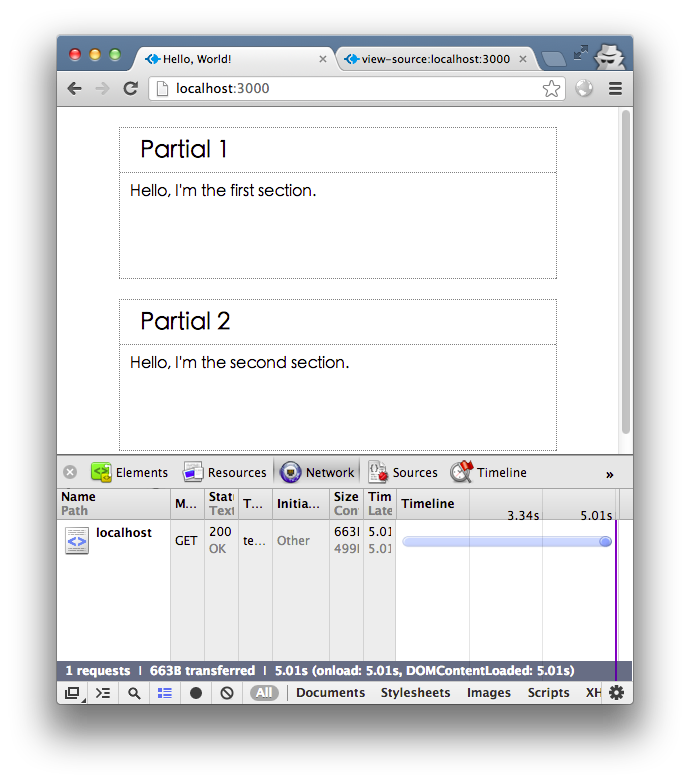``````mkdir static
cd static
curl http://code.jquery.com/jquery-1.8.3.min.js -o jquery.js
``````

``````head
title Hello, World!
script(src="/static/jquery.js")
``````

``````var static = express.static(path.join(__dirname, 'static'))
app.use('/static', function (req, res, next) {
setTimeout(static, 2000, req, res, next)
})
``````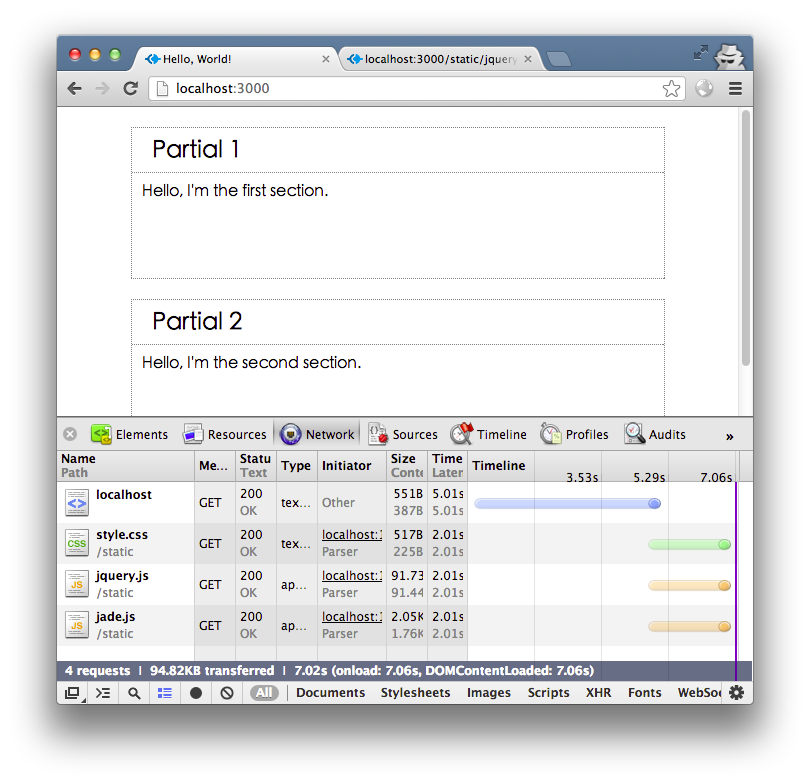``````section#s1!=s1
section#s2!=s2
``````

``````app.use(function (req, res) {
res.render('layout', function (err, str) {
if (err) return res.req.next(err)
res.write(str)
})
var n = 2
getData.d1(function (err, s1data) {
res.write('<section id="s1">' + temp.s1(s1data) + '</section>')
--n || res.end()
})
getData.d2(function (err, s2data) {
res.write('<section id="s2">' + temp.s2(s2data) + '</section>')
--n || res.end()
})
})
``````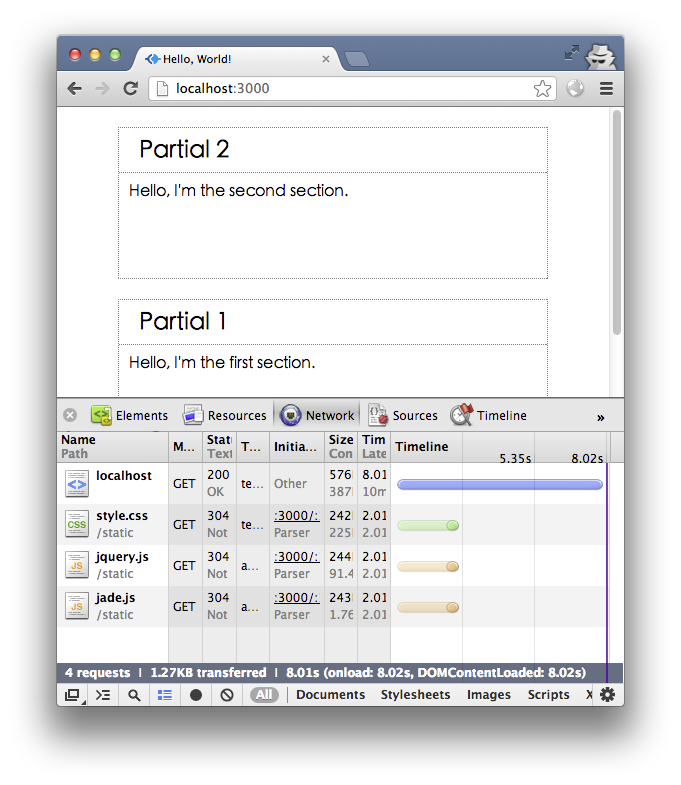``````res.render('layout', function (err, str) {
if (err) return res.req.next(err)
res.write(str)
res.write('<section id="s1"></section><section id="s2"></section>')
})
``````

``````getData.d1(function (err, s1data) {
res.write('<script>\$("#s1").html("' + temp.s1(s1data).replace(/"/g, '\\"') + '")</script>')
--n || res.end()
})
``````

s2 的处理与此类似。这时你会看到，请求网页的第二秒，出现两个空白虚线框，第五秒，出现 Partial 2 部分，第八秒，出现 Partial 1 部分，网页请求完成。

``````h1 Partial 1
.content!=content
script
``````

``````res.write('<script>\$("#s1").html("' + temp.s1(s1data).replace(/"/g, '\\"').replace(/<\/script>/g, '<\\/script>') + '")</script>')
``````

``````var resProto = require('express/lib/response')
resProto.pipe = function (selector, html, replace) {
this.write('<script>' + '\$("' + selector + '").' +
(replace === true ? 'replaceWith' : 'html') +
'("' + html.replace(/"/g, '\\"').replace(/<\/script>/g, '<\\/script>') +
'")</script>')
}
function PipeName (res, name) {
res.pipeCount = res.pipeCount || 0
res.pipeMap = res.pipeMap || {}
if (res.pipeMap[name]) return
res.pipeCount++
res.pipeMap[name] = this.id = ['pipe', Math.random().toString().substring(2), (new Date()).valueOf()].join('_')
this.res = res
this.name = name
}
resProto.pipeName = function (name) {
return new PipeName(this, name)
}
resProto.pipeLayout = function (view, options) {
var res = this
Object.keys(options).forEach(function (key) {
if (options[key] instanceof PipeName) options[key] = '<span id="' + options[key].id + '"></span>'
})
res.render(view, options, function (err, str) {
if (err) return res.req.next(err)
res.write(str)
if (!res.pipeCount) res.end()
})
}
resProto.pipePartial = function (name, view, options) {
var res = this
res.render(view, options, function (err, str) {
if (err) return res.req.next(err)
res.pipe('#'+res.pipeMap[name], str, true)
--res.pipeCount || res.end()
})
}
app.get('/', function (req, res) {
res.pipeLayout('layout', {
s1: res.pipeName('s1name')
, s2: res.pipeName('s2name')
})
getData.d1(function (err, s1data) {
res.pipePartial('s1name', 's1', s1data)
})
getData.d2(function (err, s2data) {
res.pipePartial('s2name', 's2', s2data)
})
})
``````

``````section#s1!=s1
section#s2!=s2
``````

You Can Speak "Hi" to Me in Those Ways